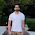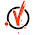## Friday, 14 December 2018

### Easiest and most effective way of detection abnormality/ outlier in time-series data

We have read many blogs on various anomaly detection algorithms. Many a times, we don't need any algorithm to detect abnormality in a system.

Different machine learning approaches to detect abnormality in system .

data scientists are using muti-angle PCA to auto-encoders to detect abnormality in a time series data. There are other complex techniques like ABOD ;used in high dimensional data and CBOF ; used when density based algorithms fail. These techniques are effective only if you know the properties of expected abnormality in system.

The most effective approach as mentioned in Anomaly detection approaches , is building an expected rule from the variables involved and any deviation form this rule is indication of abnormality in time series. One can use auto encoder , PCA or regression to build such rules. We are using regression so that audience understand the concept and don't get bogged down by related algorithms.

We can take any home appliance for example like Electric Fan. Let's say we know the temperature of fan's motor and current going into it.

from sklearn import datasets, linear_model
import matplotlib.pyplot as plt
import pandas as pd
import numpy as np

# take data values from normal running scenario, hopefully there is no issue in motor now. Generally this is the time when fan is just installed -

# creating a dummy data
data = [[352,88],[350,90],[350,89],[400,95],[400,94], [390,92], [400,93], [352,88],[350,90],[352,91],[400,95],[400,94], [390,92], [400,93],[350,90],[350,89],[400,95]]

df = pd.DataFrame(data,columns=['Current','Temp'],dtype=float)

# taking independent ( current)  and dependent variable ( temperature)  for relation ( to build using regression )
X= df['Current']
X1= X.values.reshape(X.size,1)
Y= df['Temp']
Y1 = Y.values.reshape(Y.size,1)

# fitting the regression model
regr = linear_model.LinearRegression()
regr.fit(X1, Y1)
predictions =regr.predict(X1)

# plotting error and analyzing  it
error =Y1- predictions
plt.plot(error)

plot shows that values are lying randomly between y=0 and error is in between +- 1.5. Seems a good fit. Thus we get a relation between current and temperature of motor. If we know the actual current, we can predict temperature with some accuracy. Now the concept is - 'if actual temp is far more than what it should be ( predicted from current values), then there might be some thermal abnormality in the motor. Lets extend our example further-

Taking run time data( run time values of current and temperature) from fan now;

test_X= np.array([400,380,370,355, 370,370,350, 360, 355,352,350,350,400,400,390,400,400,380,400,380,390,400,350,350])
test_Y= np.array([96,94,93, 92, 93,98,97, 98,97,88,90,89,95,94,92,94,96,94,96,93,92,94,90,90])

# predicting temperature for the present values of current ( at run time)
test_X1= test_X.reshape(test_X.size,1)
test_Y1 = test_Y.reshape(test_Y.size,1)
run_time_predictions =regr.predict(test_X1)

# plotting the errors
plt.plot(test_Y1- run_time_predictions)

Error seems high for few minutes ( between 5 to 9) . Lets combine both test and train error values.-

# combining train and test errors to include longer period of time in analysis
X_values= np.concatenate((X1, test_X1), axis=0)
Y_values= np.concatenate((Y1, test_Y1))
prections_values= np.concatenate((predictions,run_time_predictions))
Error_values= Y_values- prections_values
plt.plot(Error_values)

Y values( errors) near x=20, shows that temperature is far more that expected for specific amount of current flow. This has to be investigated further. ( coolant might not be working, spark is happening etc) . After 23-24, motor is running fine again as error is randomly distributed along y=0.

Thus at run time high error ( positive, ie. actual more than expected) is an indicator of abnormal system. I don't know how it came like somebody is showing middle finger, but exactly the middle finger is abnormal here. haha!!

The Github link for the same is present at - Python_Regression_Anomaly_Detection

1.1) Did you looked at correlation value between independent and dependent variable before applying linear regression
2) what is confidence interval coming for your parameters? How much certain you are about forecasted value becuase error plot will be directly be related to it.

1.1) no, but the co- relation between independent variables is checked.
2) confidence interval is acceptable and has to be verified by domain expert.

2.This comment has been removed by the author.

3.Very useful information about the detection abnormality/ outlier in time-series data.

Interactive Streaming Artificial Intelligence Platform RIS PACS

4.LifeVoxel.AI platform helps imaging diagnostic centers and hospitals to save up to 50%+ over conventional RIS PACS with higher functionality. LifeVoxel.AI is the fastest RIS PACS available globally and have unimaginable capabilities of centralized PACS across all your network of Imaging Centers to single window HUB.

RIS PACS
RIS PACS software